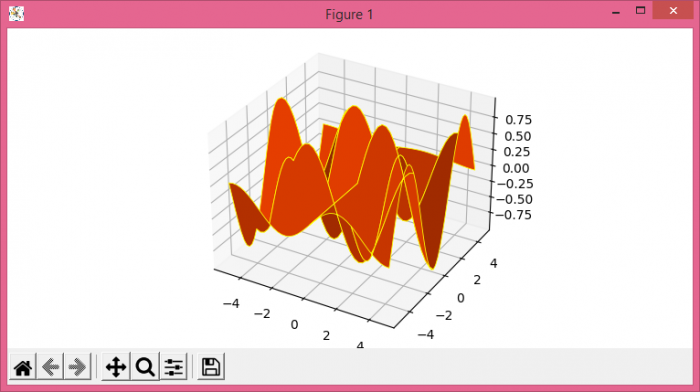# How to change the color and add grid lines to a Python Matplotlib surface plot?

MatplotlibPythonData Visualization

To change the color and add grid lines to a Python surface plot, we can take the following steps −

• Set the figure size and adjust the padding between and around the subplots.

• Create x, y and h data points using numpy.

• Create a new figure or activate an existing figure.

• Get 3D axes object, with figure (from Step 3).

• Create a surface plot, with orange color, edgecolors and linewidth.

## Example

import numpy as np
import matplotlib.pyplot as plt
from mpl_toolkits.mplot3d import Axes3D

plt.rcParams["figure.figsize"] = [7.50, 3.50]
plt.rcParams["figure.autolayout"] = True

x = np.arange(-5, 5, 0.25)
y = np.arange(-5, 5, 0.25)

x, y = np.meshgrid(x, y)
h = np.sin(x)*np.cos(y)

fig = plt.figure()

ax = Axes3D(fig)
ax.plot_surface(x, y, h, rstride=10, cstride=10, color='orangered', edgecolors='yellow', lw=0.6)

plt.show()

## Output